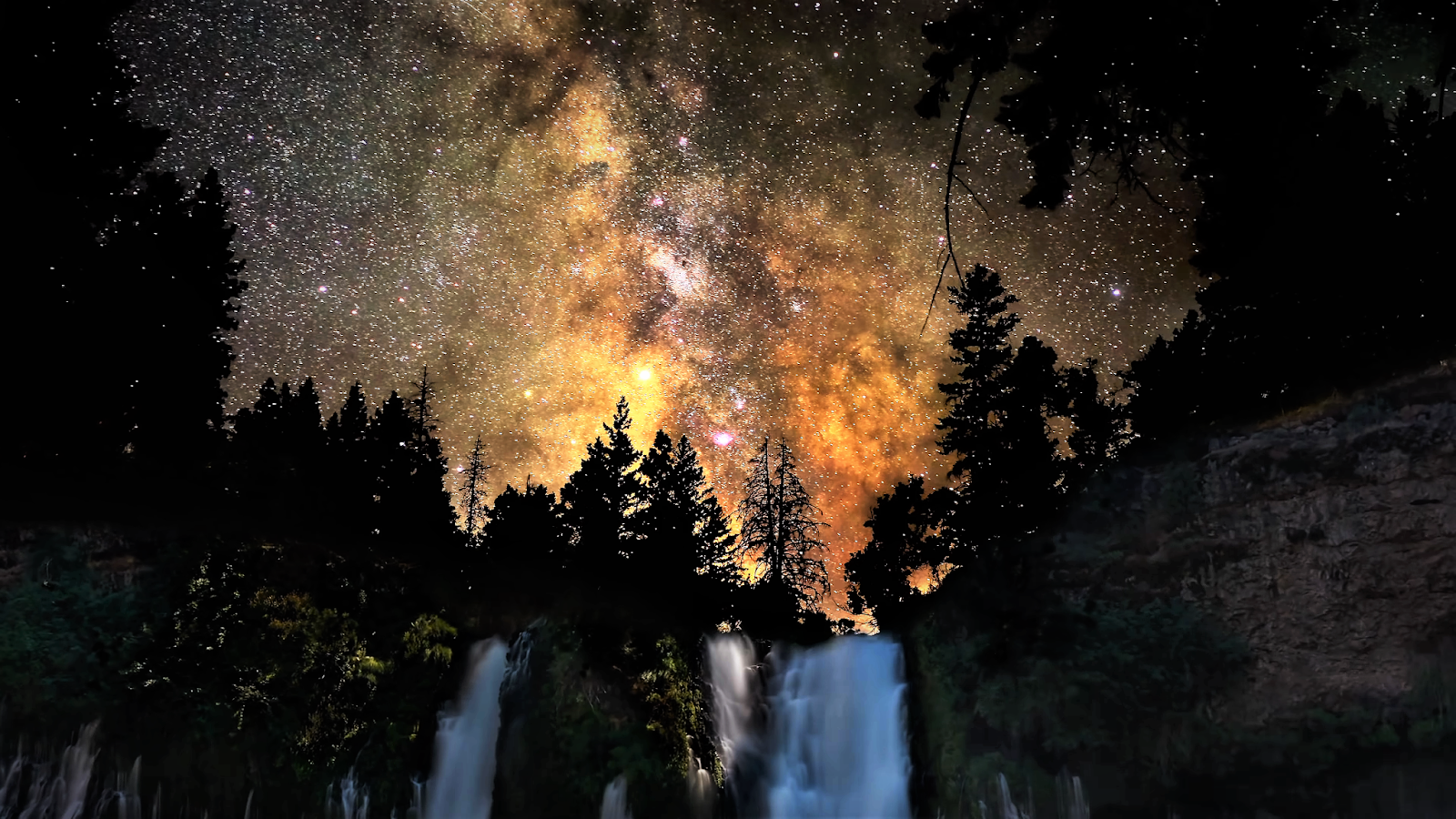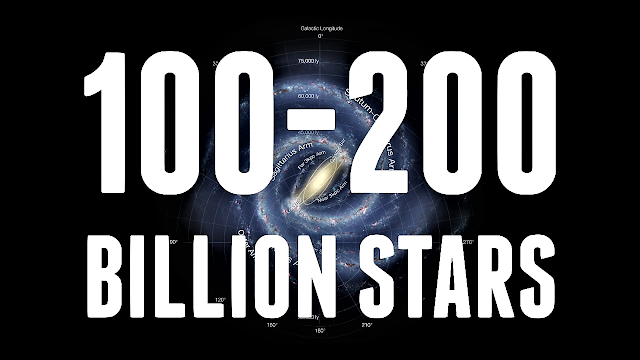Skip to main content

### How do you calculate the number of stars in the Milky Way?How many stars in the Milky Way?

🔹 The answers to the questions of science are often complex. In many cases, there aren't even any answers at all.

Knowing the exact number of stars in our galaxy, the Milky Way, is one of those questions that all experts would aim to solve in the same way: "we don't know." It's the most honest answer.

If on a night with clear skies, far from light pollution, counting the stars thinking of finding a single number would be crazy, how can we ask for the total in a galaxy?How Many Stars Are There.

"It would be like knowing precisely how many grains of sand the beaches have," says astrophysicist Santiago Vargas, a research professor at the National University's Astronomical Observatory.

He adds that even with the most sophisticated telescopes we can only see a fraction of that large number of stars in the Milky Way, a galaxy so enormous that a ray of light would take 100,000 years to cross from one end to the other.

However, scientists have tried to give an idea of how many stars there would be in the Milky Way. Astrophysicist Germán Chaparro, research professor at Ecci University, explains that the mass of our galaxy is calculated and then the percentage of mass that the stars are made of.

#### How many stars are in our galaxy?

Hence, the most common answers suggest that it would be about 100 billion, if we take as a base stars larger or smaller than our Sun and make an average. But if the estimate is made with a different amount of mass, the number rises to 400 billion.

"We know the mass of stars because of the light that reaches us, the colour, luminosity and chemical composition. We take that light and with physical processes that have been developed in the last 80 years it is characterized. That's how we know how old they are," says Chaparro about the question of how many stars are in our galaxy? (Milky Way) .

This experiment was carried out with the star Kepler 452 - orbited by the planet Kepler 452b, the 'cousin' of the Earth - and thus it was possible to know that it is 6 billion years old.

There are other models for estimating the number of stars in our galaxy, but in the end the answers will depend on what average mass of a star is used.How many stars are in our galaxy?